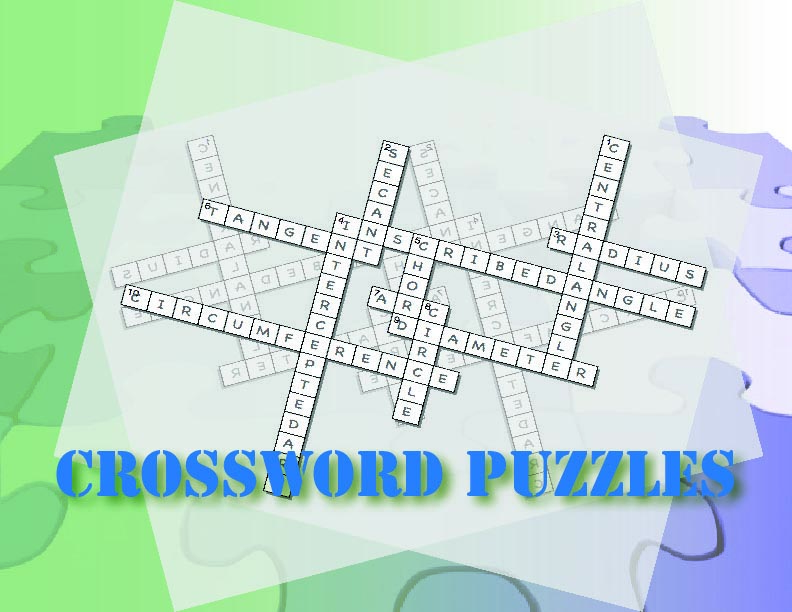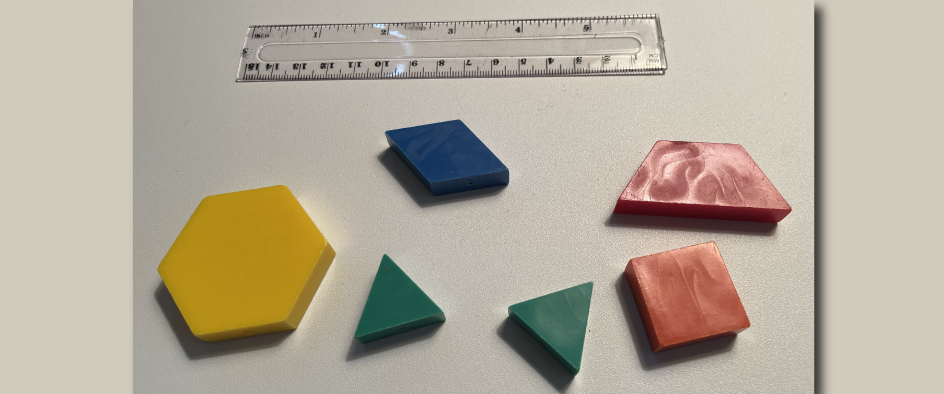# How Media4Math Can Transform Your Math Instruction## Units of Study Will Include

 Counting and Skip-Counting Repeated Addition and Multiplication Fractions Decimals Ratios, Proportions and Percents Equations Functions and Relations Variables, Constants, and Unknowns Linear Functions Quadratic Functions Geometry Basics: Points and Lines Geometry Basics: Angles and Planes Geometry Basics: Triangles Geometry Basics: Quadrilaterals Geometry Basics: Polygons Geometry Basics: Circles

## Sample Unit: Equations

### The suggested timeframe for each lesson can be adapted to suit your needs.### Days 1-2: Speaking Math

Introduction of key vocabulary around equations. This is a gamified experience with word puzzles and other games.

Introduce these terms and definitions

• Equation
• Equality
• Solution
• True Equation
• False Equation
• Algebraic Expression
• Equals Sign
• Conditional Equation
• The Unknown

Media4Math Resources:

Goal: A low-stress intro to equations vocabulary. These two days lay the groundwork of vocabulary and basic concepts. This also reinforces the notion that math is a language, an understandable one that students can comprehend.### Days 3-4: Building Blocks

Introduction to the properties of equality. Use the Equation Game to explore:

• Addition Property of Equality
• Subtraction Property of Equality
• Multiplication Property of Equality
• Division Property of Equality

Media4Math Resources:

Goal: To develop a concrete understanding of equations and the properties of equality. Establishes that math is not just computation, but logical reasoning.### Days 5-6: Putting it Together

Watch instructional videos on equations and solving one- and two-step equations.

Pre-viewing Questions

• Do you know how to solve an equation?
• What is the process for solving an equation?
• What does it mean to have a solution to an equation?

Post-viewing Questions

• What does it mean to isolate the variable?
• How does solving an equation change a conditional equation?

Media4Math Resources:

Goal: Develop mastery at solving one- and two-step equations using properties of operations.### Days 7-8: Show What You Know

This student module includes assessment items. You can assign this module to individual students and create a dashboard to track student progress.

Media4Math Resources:

Goal: Assess student mastery.

## Did you know that with the Media4Math bundle subscription you get access to all these resources? Want to learn more, Contact Us.

 Sign Up for a Free Account!   Become a part of the Media4Math community. Get our monthly newsletter. Save our free resources in your MyContent folder. Learn how to incorporate digital resources into your instruction.